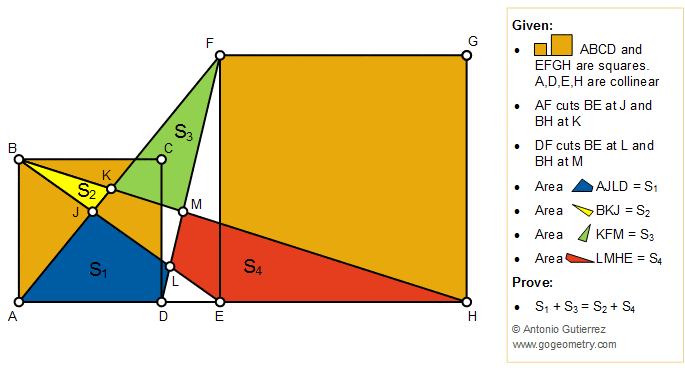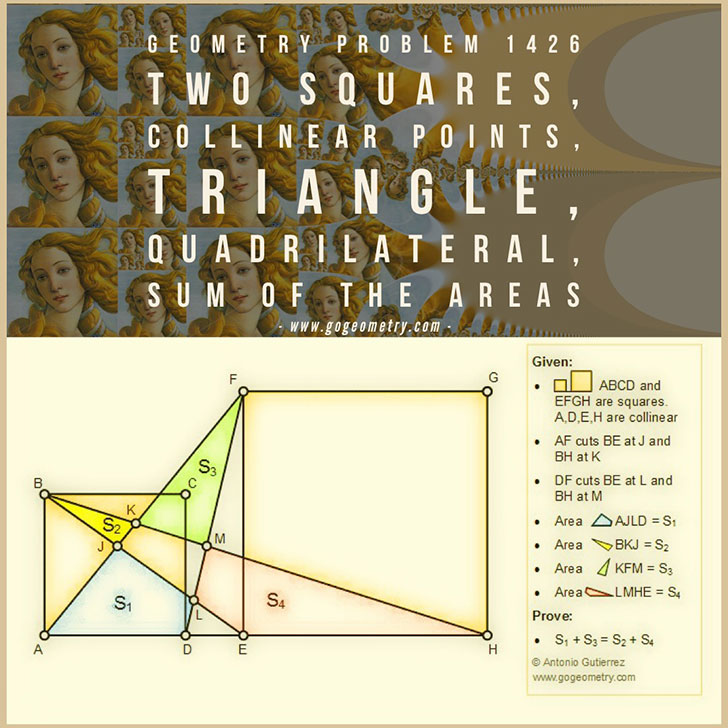Geometry Problem 1426: Two Squares, Collinear Points, Triangle, Quadrilateral, Sum of the Areas

The figure below shows two squares ABCD and EFGH so that A, D, E, and H are collinear points. Line AF cuts BE at J and BH at K, Line DF cuts BE at L and BH at M. If area AJLD = S1, area BKJ = S2, area KFM = S3, and area LMHE = S4, prove that S1 + S3 = S2 + S4.Geometric Art of Problem 1426: Sketching, Typography, iPad AppsAnimation of the Conformal Mapping or Transformation of Problem 1426Conformal Mapping

A conformal mapping or conformal transformation is a continuous mapping preserving the form of infinitesimal figures. This conformal map produces a realistic view of the original image or map. This the conformal transformation of problem1426